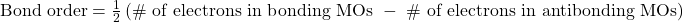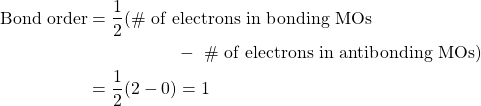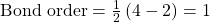Chapter 9. Chemical Bonds

# Molecular Orbitals

Jessie A. Key

Learning Objectives

1. Gain an  understanding of molecular orbital theory.
2. Learn to calculate bond orders.
3. Learn to draw molecular orbital electron configuration energy diagrams.

Valence bond theory is able to explain many aspects of bonding, but not all. To complement this theory, we use another called the molecular orbital (MO) theory. Molecular orbital theory is a more sophisticated model for understanding the nature of chemical bonding.

MO theory takes the idea of atomic orbitals overlapping to a new level, where new molecular orbitals are generated using a mathematical process called linear combination of atomic orbitals (LCAO).

Molecular orbitals share many similarities with atomic orbitals:

• They are filled from lowest energy to highest energy (Aufbau principle).
• They can hold a maximum of two electrons of opposite spin per orbital (Pauli exclusion principle).

The major difference between atomic and molecular orbitals is that atomic orbitals represent electron density in space associated with a particular atom. Molecular orbitals are associated with the entire molecule, meaning the electron density is delocalized (spread out) over more than one atom.

# The Molecular Orbitals of the Hydrogen Molecule

Combining the 1s orbitals of each hydrogen atom using LCAO, two molecular orbitals are generated σ1s (pronounced sigma one s) and σ*1s (pronounced sigma star one s).

The σ1s orbital is generated by a constructive combination (or interference), where the two atomic orbitals wave functions reinforce (add to) each other. This is the lower energy of the two molecular orbitals and is known as the bonding molecular orbital. Notice in Figure 9.19 “Hydrogen molecular orbital combination diagram” that the electron density of this orbital is concentrated between the two nuclei. These electrons are stabilized by attractions to both nuclei, and they hold the atoms together with a covalent bond.

The σ*1s orbital is generated by a destructive combination (or interference), where the wave functions of the two atomic orbitals cancel each other. This type of combination results in an area of zero electron density between the two nuclei, known as a nodal plane (or node). This node of zero electron density is destabilizing toward the bond, making it higher energy, and subsequently this type of orbital is known as an antibonding molecular orbital (denoted by the asterisk in the orbital name).

Similar to atomic orbitals, we can write electron configuration energy diagrams for molecular orbitals (Figure 9.20 “Hydrogen molecular orbital electron configuration energy diagram”). Notice that the atomic orbitals of each atom are written on either side, and the newly formed molecular orbitals are written in the centre of the diagram. The bonding molecular orbital is filled and is relatively lower in energy than the contributing atomic orbitals, supporting the fact that hydrogen molecules (H2) are more stable than lone hydrogen atoms.

# Bond Order

We have just seen that the bonding molecular orbital is lower energy and promotes the formation of a covalent bond, while the antibonding molecular orbital is higher energy with a node of zero electron density between the atoms that destabilizes the formation of a covalent bond. We can evaluate the strength of a covalent bond by determining its bond order.Bond-order values can be whole numbers, fractions, or zero. These values correspond to the valence bond model, so a bond order of 1 is equal to a single bond, and 2 is equal to a double bond. A value of zero means that there is no bond present, and the atoms exist separately.

Example 9.11

Determine the bond order of the hydrogen molecule.

SolutionTherefore there is a single bond in the hydrogen molecule.

# Molecular Orbitals of Li2

Generating molecular orbitals of molecules more complex than hydrogen using the LCAO method requires following a few additional guidelines:

• The number of MOs generated is equal to the number of atomic orbitals combined.
• Combined atomic orbitals should be of similar energy levels.
• The effectiveness of atomic orbital combination depends on the amount of orbital overlap. Increased overlap lowers the energy of the bonding molecular orbital further, and raises the energy of the antibonding molecular orbital.

Let’s follow these guidelines and generate a molecular orbital electron configuration diagram for Li(Figure 9.21 “Molecular orbital electron configuration energy diagram for dilithium”):

Notice that we have combined the 1s atomic orbitals, as before in the H2 example, to generate bonding and antibonding molecular orbitals that are completely filled by both atoms’ 1s electrons. Similarly 2s atomic orbitals combine, giving a bonding orbital and an antibonding orbital, which are filled with the remaining valence electrons starting from the bottom up. The atomic orbitals that combine are of similar energy levels; a 1s orbital does not combine with one of the 2s orbitals.

The bond order can be determined for this molecule to be:Therefore Li2 would have a single bond.

# Molecular Orbitals from p Atomic Orbitals

To determine the molecular orbitals of many other molecules, we need to examine how p orbitals combine to give molecular orbitals. The p orbitals can overlap in two ways: head-to-head or sideways. Head-to-head overlap of p atomic orbitals results in a bonding and antibonding molecular orbital, where the electron density is centred along the internuclear axis, making them σ orbitals (Figure 9.22 “Head-to-head overlap of p orbitals”).

Sideways overlap of the remaining four p atomic orbitals can occur along the two other axes, generating four π molecular orbitals having electron density on opposite sides of the internuclear axis (Figure 9.23 “Sideways overlap of p orbitals”).

The head-to-head overlap giving σ molecular orbitals results in greater overlap, making its bonding molecular orbital the most stable and lowest energy, while the σ* antibonding is least stable and has the highest energy (Figure 9.24 “Molecular orbital energy diagram for homonuclear diatomic molecules made from atoms of atomic number 8-10”). Sideways overlap gives four π molecular orbitals, two lower-energy degenerate-bonding molecular orbitals, and two higher-energy antibonding orbitals.

The energy diagram we have just generated fits experimentally with O2, F2, and Ne2, but does not fit for B2, C2, and N2. In the latter, homonuclear diatomic molecules (B2, C2, and N2), interactions take place between the 2s and 2p atomic orbitals that are strong enough to swap the ordering of the σ2p and π2p molecular orbitals (Figure 9.25).

# Heteronuclear Diatomic Molecules

In heteronuclear diatomic molecules, where two different molecules are bonded, the energy levels of the individual atoms’ atomic orbitals may differ. However, the molecular orbital diagram we see in Figure 9.25 (“Molecular orbital energy diagram for homonuclear diatomic molecules made from atoms of atomic number 5-7”) can be used to estimate the electron configuration and bond order.

# Frontier Molecular Orbitals

We can focus further on two very important types of molecular orbitals: the highest occupied molecular orbital (HOMO) and the lowest unoccupied molecular orbital (LUMO), also referred to collectively as the frontier molecular orbitals (Figure 9.26 “Frontier molecular orbitals HOMO and LUMO”). As their names imply, the HOMO is the molecular orbital that has the highest energy and contains electrons, while the LUMO is the lowest energy molecular orbital that does not contain electrons.

When molecules absorb energy, it is typical for a HOMO electron to use this energy to transition from the ground HOMO orbital to the LUMO excited-state orbital. This type of transition can be observed in ultraviolet-visible (UV-Vis) radiation spectroscopy experiments. As well, in many chemical reactions, one reactant molecule may donate HOMO electrons to the LUMO of another reactant (Figure 9.27 “Formation of a new bonding molecular orbital by combining reactant HOMO and LUMO”). Therefore, understanding frontier molecular orbital energy levels can provide chemists with a great deal of insight in the areas of molecular spectroscopy and reactivity.

Key Takeaways

• Atomic orbitals can combine to make bonding and antibonding molecular orbitals.
• Bonding orbitals are lower in energy than antibonding orbitals.
• Molecular orbitals are filled using similar principles to atomic orbitals.
• Bond order can be used to evaluate bond strength.
• Frontier molecular orbitals are of particular importance in molecular spectroscopy and reactivity.